###### Define the law of variable proportion.

The law of variable proportions examines the production with one fac variable, keeping the quantities of other factors fixed. When the quantity of one factor is changed, keeping the quantity of other factors constant, the proportion between the variable factor and fixed factor is changed. Therefore, it is known as law variable proportions.

###### What are the causes of increasing returns in the first stage?

The causes of increasing returns in the first stage are as follows:
i. Increase in efficiency of fixed factor
ii. Increase in the efficiency or productivity of variable factor

###### What are the causes of diminishing returns in the second stage?

The causes of decreasing returns in the second are as follows:
i. Scarcity of fixed factor
ii. Indivisibility of fixed factor
iii. Imperfect substitutability of the factor

###### What are the causes of negative returns in the third stage?

As the amount of the variable factor continuous to be increases to the constant quantity of the other, a stage is reached when the total product declines & the marginal product becomes negative. A return to factors refers to the effects on total output of changes in one factor alone with other factors being held as fixed.

###### What is the marginal product when total product when total product is constant?

When total product is constant, marginal product will be zero.

###### Which stage of production is chosen by a rational producer in the short run?

A rational producer chooses to produce in the second stage where both MP and AP of variable factor are diminishing. In this stage, the producer will decide to produce depending upon the prices of factors.

###### State the behaviour of TP, AP and MP in 2nd stage of production under Law of Variable Proportion.

In the second stage of law of variable proportion TP increases at the decreasing rate; AP continuously decreases and MP also continuously decreases. When TP becomes maximum, MP becomes zero.

###### What is iso-quant?

Iso-quant is defined as the locus of different combinations of any two inputs that yields the same level of output. It is downward slopping and convex to the origin.

###### What is production function? Explain its types. Or, Define production function. Explain short run and long run production function. Or, Explain the concept of production function.

Production function is the functional relationship between factor inputs and output under given technology. In its general form, it tells that production of a commodity depends on certain specific inputs. In its specific form, it presents the quantitative relationship between inputs & output. Besides, the production function represents the technology of a firm, an industry or an economy as a whole. A production function may take the form of a schedule or table, a graphed line or curve, an algebraic equation or a mathematical model. But each of these forms of a production function can be converted into its other forms.
The long-run production function is generally expressed as Q =f(L, L, K, M, T, …)
where
Lb = Land & building
L = Labour
K= Capital
M= Materials
T = Technology
f = Function relationship
For the sake of convenience and simplicity, we consider production to be dependent on the amount of two variable factors. It is expressed as:
Q = f(L,K)
Types of Production Function
There can two kinds of production function:
i. Short-run production functions: Short-run is the time period that a firm change all of its input factors. In the short-run, some factors of production like land, factory size, machinery etc. remain constant or fixed but some factors of production like labour, fuel, raw material etc. are variable. Thus, the short-run production function is the technical or mathematical relationship between inputs and output when quantities of some inputs are kept constant and quantities of one input is varied. The input and output relationship in the short-run is studied under the law of variable proportions. This law relates to the short-run production function because only one or few factors of production are varied in the short-run. It is also known as the production function with one variable input. It can be expressed as
Q=f(L, K’)
Where, labour (L) is variable factor and capital (K’) is fixed factor.
ii. Long-run production functions: Long-run is the time period that a firm can change all of its input factors. It means that in the long-run all factors of production are variable or no factor of production is fixed. Thus, the long-run production function is the technical or mathematical relationship between inputs and output when all inputs or factors of production are variable. The input and output relationship in the long-run is studied under the laws of returns to scale. It is also known as the production function with two variable inputs. It can be expressed as
Q = f(L,K)
Where, both labour (L) and capital (K) are variable.

###### Explain the method of least cost combination of factors or maximizing level of output for a given level of outlay or cost. Or, What is producer’s equilibrium? How does producer attain equilibrium under given total cost outlay?

A producer is said to be in equilibrium when he is producing the desired output at the least possible cost. It is also known as the least cost combination of inputs or factors of production.
An iso-quant map represents the various factor combinations which can yield various level of output. Every iso-quant shows those factor combinations each of which can produce a specified level of output. Thus, an iso-quant map represents the production function of a product with two variable factors. Therefore, an iso-quant map represents the technical conditions of production for a product. On the other hand, a family of iso-cost map represents the various levels of total cost or outlay given the price of two factors. A producer may desire to he may desire to maximize his output level for a given cost.
Conditions for equilibrium
The producer will be in equilibrium when the following two conditions are fulfilled:
i. First order (necessary) condition: Iso-cost line is tangent to the isoquant.
ie. Slope of IQ = Slope of Iso-cost line
or, -MRTSLK = – w/r
or, MPL/MPK =W/r
where
W = wages
r=rate interest
MPL = Marginal productions of labour
MPK = Marginal productions of capital
ii. Second order (sufficient) condition: The isoquant must be convex to the origin. A rational producer is expected to maximize output for a given cost. Alternatively, he may attempt to minimize cost subject to a given level of output. In this section, we will explain how a producer maximizes his output for a given cost. Suppose, a producer has decided upon an outlay, it has to incur for the production of a commodity. With a given level of outlay, there will be a single (so-cost line that represents the outlay that firm has decided to spend. The firm will have to choose a factor combination lying on the given iso-cost line.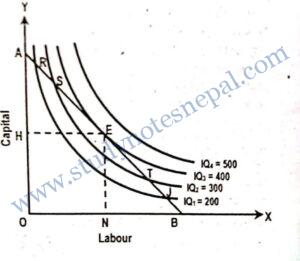Obviously, with a given cost or outlay, a rational producer will be interested in maximizing output of the commodity.
Suppose a producer has decided to incur an outlay of Rs.500 on labor & capital which is represented by the iso-cost line AB. A producer has a choice to use any factor combination of labor & capital such as R, S E, and T & J lying on the given iso-cost line AB to produce the product. An iso-quant maps showing a set of iso-quant curve that represents various level of output (200,300,400,500 units) has been superimposed on the given iso-cost line AB. In the fig. a producer will choose the factor combination E consisting of ON of labor & OH of capital. This is because of all the factors combinations that lie on the given iso cost line AB, only the factor combination E enables the producer to reach the higher possible iso-quant IQ3 and thus produce 400 units of output. All other combinations of labor & capital that lie on the given iso-cost line AB such as R, S, T & J lie on lower iso-quant showing lower level of output.

###### Explain the law of variable proportion. Or, State and explain the short run production.

The law of variable proportion is a very important law of economics. This law was developed by Alfred Marshal, Benham, Samuelson, and Robinson. It is concerned with short-run production function. production with one variable input, keeping other inputs fixed. When the This law examines the quantity of one factor is changed, keeping the quantity of other factors constant, the proportion between the variable factor and fixed factor is changed. Therefore, it is known as law of variable proportion.

According to this law, as the quantity of a variable factor is increased by equal amount keeping the quantities of other factors constant, initially total product increases at an increasing rate, but after a point increases at a diminishing rate, becomes maximum and starts to decline.

The assumptions of the law of variable proportions are:

1. The state of technology is constant.
2. At least one factor of production is fixed.
3. There is possibility of varying the proportion of factors of production. On the basis of the above mentioned assumptions, the law of variable proportion can be explained with the help of the table and figure.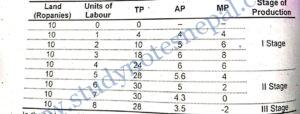In the table, land is a fixed factor and labour is a variable factor. With a fixed quantity of land as a farmer raises employment of labour from one unit to 6th unit, total product increases from 1 unit to 6th unit and become maximum at the 7th unit of labour after then TP starts to decline. This fact can be viewed clearly from MP column. MP up to 3rd unit of labour increases and starts to diminish. At 7th unit of labour, it is zero and then it is negative. Hence, TP increases at an increasing rate up to 3rd unit of labour and then increases at a decreasing rate up to 6th unit of labour. It becomes maximum at the 7th unit of labour after then diminishes.

The average product reaches maximum at the 4th unit of labour i.e. one step later than MP after this AP starts to diminish.

The law of variable proportions is presented diagrammatically as below.

The law of variable proportions can be divided into three stages. These three stages of short-run law of production are explained below.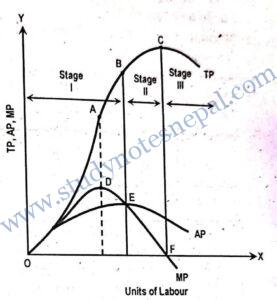1. Stage I (Increasing returns): In this stage, total product increases at an increasing rate to a certain point and then rate of increasing switches from increasing to diminishing. In the fig., TP is increasing up to point A at an increasing rate and then has started increasing at diminishing rate. The average product increases throughout this stage and reaches to maximum at point E. The boundary of this stage is point E where marginal and average products are equal.
2. Stage II (Decreasing returns): In this stage, total product continues to increase at a diminishing rate until it reaches its maximum point at C where second stage ends. In this stage, both the marginal product and average product are diminishing but are positive. At the end of second stage, i.e. at point F marginal product is zero.
3. Stage III (Negative returns): This stage starts from the point C. In this stage total product is declining and marginal product is negative and marginal product curve MP goes below the x-axis. In this stage, variable factor is too much relative to the fixed factor.
###### Explain and illustrate the concept of isoquant.

An isoquant is the locus of different combinations of two inputs (labour and capital) yielding the same level of output. The given quantity of a commodity can be produced by the different combinations of two inputs. The term ‘iso -quant’ has been derived from a Greek word ‘iso’ meaning equal and Latin word ‘quant’ meaning quantity. Therefore, the iso-quant curve is also known as equal product curve and production indifference curve.

Isoquant is based on the following assumptions:

i.There are only two inputs (L.K) to produce a good. it.

ii.The two inputs (L&K) can be substituted for one another at a diminishing up to a certain limit.

iii Production function is continuous. It means labour and capital perfectly divisible.

On the basis of these assumptions, isoquant can be explained by the help of following table: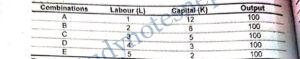The table shows different combinations A, B, C, D and E which can produce 100 units of X good. Combination A consists of 1 unit of labor and 12 units capital. Similarly Combination B consists of 2 units of labor and 8 units of capital. Combinations C consist of 3 units of L & 5 units of K, combinations D consists & 4 units of L & 3 units of K, and domination E consists of 5 units of L & 2 units of K. All these combinations of inputs produce the same level of output, i.e. 100 units.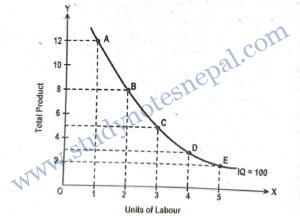In the figure, points A, B, C, D and E on the iso-quant IQ show five different combinations of capital and labour. But all these combinations yield the same output i.e. 100 units. Movement from A to E indicates decreasing quantity of capital and increasing quantity of labour. This implies substitution of labour for capital yields the same level of output whatever be the combination of capital & labor.